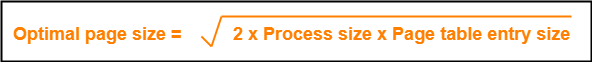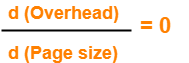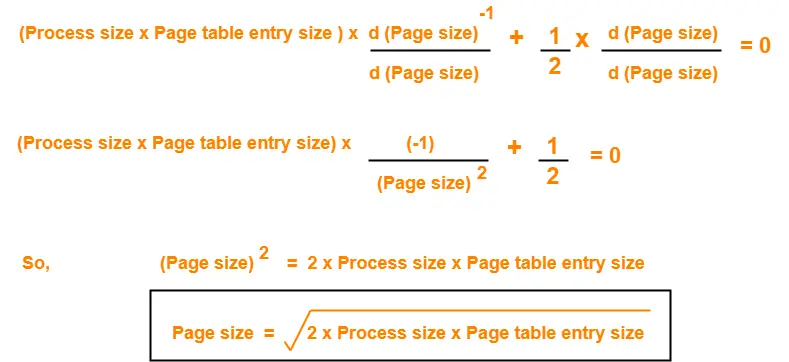# Optimal Page Size | Paging

## Paging in OS-

Before you go through this article, make sure that you have gone through the previous article on Paging in OS.

We have discussed-

• Paging is a non-contiguous memory allocation technique.
• Page Table keeps track of the frames storing the pages of the process.

In paging scheme, there are mainly two overheads-

### 1. Overhead of Page Tables-

• Paging requires each process to maintain a page table.
• So, there is an overhead of maintaining a page table for each process.

### 2. Overhead of Wasting Pages-

• There is an overhead of wasting last page of each process if it is not completely filled.
• On an average, half page is wasted for each process.

Thus,

= Size of its page table + (Page size / 2)

## Optimal Page Size-

Optimal page size is the page size that minimizes the total overhead.

It is given as-Also Read- Important Formulas Of Paging

## Proof-

Total overhead due to one process

= Size of its page table + (Page size / 2)

= Number of entries x Page table entry size + (Page size / 2)

= Number of pages the process is divided x Page table entry size + (Page size / 2)

= (Process size / Page size) x Page table entry size + (Page size / 2)

Now,Keeping process size and page table entry size as constant, differentiating overhead with respect to page size, we get-## Problem-01:

In a paging scheme, virtual address space is 4 KB and page table entry size is 8 bytes. What should be the optimal page size?

## Solution-

Given-

• Virtual address space = Process size = 4 KB
• Page table entry size = 8 bytes

We know-

Optimal page size

= (2 x Process size x Page table entry size)1/2

= (2 x 4 KB x 8 bytes)1/2

= (216 bytes x bytes)1/2

= 28 bytes

= 256 bytes

Thus, Optimal page size = 256 bytes.

## Problem-02:

In a paging scheme, virtual address space is 16 MB and page table entry size is 2 bytes. What should be the optimal page size?

## Solution-

Given-

• Virtual address space = Process size = 16 MB
• Page table entry size = 2 bytes

We know-

Optimal page size

= (2 x Process size x Page table entry size)1/2

= (2 x 16 MB x 2 bytes)1/2

= (226 bytes x bytes)1/2

= 213 bytes

= 8 KB

Thus, Optimal page size = 8 KB.

## Problem-03:

In a paging scheme, virtual address space is 256 GB and page table entry size is 32 bytes. What should be the optimal page size?

## Solution-

Given-

• Virtual address space = Process size = 256 GB
• Page table entry size = 32 bytes

We know-

Optimal page size

= (2 x Process size x Page table entry size)1/2

= (2 x 256 GB x 32 bytes)1/2

= (244 bytes x bytes)1/2

= 222 bytes

= 4 MB

Thus, Optimal page size = 4 MB.

Next Article- Practice Problems On Paging in OS

Get more notes and other study material of Operating System.

Watch video lectures by visiting our YouTube channel LearnVidFun.

SummaryArticle Name
Optimal Page Size | Paging
Description
In paging scheme, Optimal page size is the page size that minimizes the total overhead. Practice Problems based on Optimal page size. Paging in OS divides process into pages.
Author
Publisher Name
Gate Vidyalay
Publisher Logo